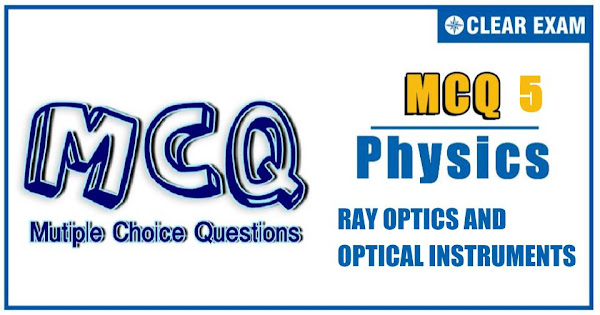## [LATEST]\$type=sticky\$show=home\$rm=0\$va=0\$count=4\$va=0

JEE Advanced Physics Syllabus can be referred by the IIT aspirants to get a detailed list of all topics that are important in cracking the entrance examination. JEE Advanced syllabus for Physics has been designed in such a way that it offers very practical and application-based learning to further make it easier for students to understand every concept or topic by correlating it with day-to-day experiences. In comparison to the other two subjects, the syllabus of JEE Advanced for physics is developed in such a way so as to test the deep understanding and application of concepts.

Q1. A fish is vertically below a flying bird moving vertically down toward water surface. The bird will appear to the fish to be
•  Moving faster than its speed and also away from the real distance
•  Moving faster than its real speed and nearer than its real distance
•  Moving slower than its real speed and also nearer than its real distance
•  Moving slower than its real speed and away from the real distance
Solution
μ_1/(-u)+μ_2/v=(μ_2-μ_1)/R For a plane surface, R=∞ ∴μ_1/(-u)+μ_2/v=0 orμ_2/v-μ_1/uorμ/v-1/u orv=μ_2 u Clearly, to the fish, the bird appears farther than its actual distance Again, dv/dt=μ du/dt or Apparent speed of bird = refractive index × actual speed of bird
Q2.In a side show programme, the image on the screen has an area 900 times that of the slide. If the distance between the slide and the screen is x times the distance between the slide and the projector lens, then

•  x=30
•  x=31
•  x=500
•  x=1/30
Solution
m=30 v+u=xu Or v/u+1=xor m+1=x Or x=31
Q3.  A light ray I is incident of a plane mirror M. The mirror is rotated in the direction as shown in the figure by an arrow at frequency 9/π rps. The light reflected by the mirror is received on the wall W at a distance 10 m from the axis of rotation. When the angle of incidence becomes 37°, the speed of the spot (a point) on the wall is
•   10 ms^(-1)
•  1000 ms^(-1)
•  500 ms^(-1)
•  None of these

Q4. You are given two identical plano-convex lenses. When you place an object 20 cm to the left of a single plano-convex lens, the image appears 40 cm to the right of the lens. You then arrange the two plano-convex lenses back to back to form a double convex lens. If the object is 20 cm to the left of this new lens, what is the approximate location of the image?
•  μ>10 cm to the right of the lens
•  20 cm to the right of the lens
•  μ>80 cm to the right of the lens
•  80 cm to the left of the lens
Q5. A parallel beam of light is incident on the system of two convex lenses of focal lengths f_1=20 cm and f_2=10 cm. What should be the distance between two lenses so that rays after refraction from both the lenses pass undeviated?
•  60 cm
•  30 cm
•  90 cm
•  40 cm
Q6. A bi-convex lens is formed with two thin plano-convex lenses as shown in the figure. Refractive index n of the first lens is 1.5 and that of the second lens is 1.2. Both the curved surfaces are of the same radius of curvature R=14 cm. For this bi-convex lens, for an object distance of 40cm, the image distance will be
•  -280.0cm
•  40.0cm
• 21.5 cm
•  13.3 cm
Q7.A ray of monochromatic light is incident on the refracting face of a prism (angle 75°). It passes through the prism and is incident on the other face at the critical angle. If the refractive index of the prism is √2, then the angle of incidence on the first face of the prism is
•  15°
•  30°
•  55°
•  60°
Solution

sin⁡ ic =1/√2 ic=45° Now, 75°=r+45° Or r=30° Now, sin⁡i/(sin⁡30°)=√2 Or sin⁡i=√2×1/2=1/√2 ∴ i=45°
Q8.A point objects is placed at the centre of a glass sphere of radius 6 cm and refractive index 1.5. The distance of the virtual image from the surface of the sphere is
•  2 cm
•  T4 cm
•  6 cm
•  12 cm
Solution

When the object is placed at the center of the glass sphere, the rays from the object fall normally on the surface of the sphere and emerge undeviated.

Q9.An object is placed at a distance of 25 cm from the pole of a convex mirror and a plane mirror is set at a distance 5 cm from convex mirror so that the virtual images formed by the two mirrors do not have any parallax. The focal length of the convex mirror is
•  37.5 cm
•  -7.5 cm
•  -37.5 cm
•  +7.5 cm
Solution

Clearly, the distance of I from P is 15 cm Now, u=-25 cm, v=15 cm, f=? 1/u+1/v=1/f or 1/f=1/(-25)+1/15 Or 1/f=(-3+5)/75 or f=75/2 cm =37.5 cm
Q10. A linear object AB is placed along the axis of a concave mirror. The object is moving towards the mirror with speed U. The speed of the image of the point A is 4U and the speed of the image of B is also 4U but in opposite direction. If the center of the line AB is at a distance L from the mirror then find out the length of the object
•  3L/2
•  5L/3
•  L
• None of these#### Written by: AUTHORNAME

AUTHORDESCRIPTION## Want to know more

Please fill in the details below:

## Latest NEET Articles\$type=three\$c=3\$author=hide\$comment=hide\$rm=hide\$date=hide\$snippet=hide

Name

ltr
item
BEST NEET COACHING CENTER | BEST IIT JEE COACHING INSTITUTE | BEST NEET & IIT JEE COACHING: Ray Optics and Optical Instruments-Quiz-5
Ray Optics and Optical Instruments-Quiz-5
https://1.bp.blogspot.com/-u7taCFrAMoA/YN3tTofuUcI/AAAAAAAALuo/MY_MFQ_P4S4K1dm4zUr7Tvb3WUdl7yCuQCLcBGAsYHQ/s600/Quiz%2BImage%2B20.jpg
https://1.bp.blogspot.com/-u7taCFrAMoA/YN3tTofuUcI/AAAAAAAALuo/MY_MFQ_P4S4K1dm4zUr7Tvb3WUdl7yCuQCLcBGAsYHQ/s72-c/Quiz%2BImage%2B20.jpg
BEST NEET COACHING CENTER | BEST IIT JEE COACHING INSTITUTE | BEST NEET & IIT JEE COACHING
https://www.cleariitmedical.com/2021/07/ray-optics-and-optical-instruments-quiz_22.html
https://www.cleariitmedical.com/
https://www.cleariitmedical.com/
https://www.cleariitmedical.com/2021/07/ray-optics-and-optical-instruments-quiz_22.html
true
7783647550433378923
UTF-8

STAY CONNECTED